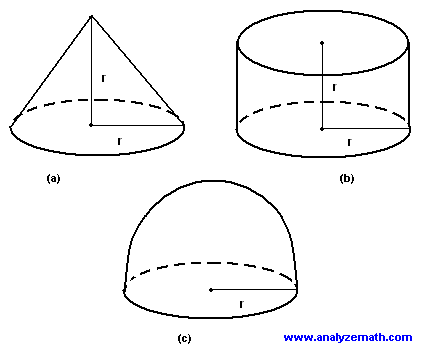# Compare Volumes of 3D shapes

A problem to compare the volumes of a cone, a cylinder and a hemisphere.

## Problem

Find and compare the volumes of the three shapes shown below: (a) A cone with height r and radius of the base r, (b) a cylinder of height r and radius of the base r and (c) a hemisphere of radius r.Solution to Problem 1:

• Volume (Vco) of the cone is give by:
Vco = (1 / 3)*area of base * height
= (1 /3) * Pi r 2 * r = (1 /3) * Pi r 3
• Volume (Vcy) of the cylinder is give by:
Vcy = area of base * height
= Pi r 2 * r = Pi r 3
• Volume (Vhe) of the hemisphere is give by:
Vhe = (1 / 2) volume of a sphere
= (1/2) (4/3) Pi r 3 = (2 / 3) Pi r 3
• The volume of the cylinder is the largest. The volume of the cone is one third of the volume of the cylinder and it is the smallest. The volume of the hemisphere is twice the volume of the cone or two thirds the volume of the cylinder.

## More References and Links to Geometry

Geometry Tutorials, Problems and Interactive Applets.